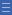## Featured resource

Home > Topdrawer > Fractions

# Fractions

Fractions

Fractions

Fractions are more difficult to understand than whole numbers because they are relational numbers. A sound understanding of fractions is essential to progress in mathematics learning.

Students' first experiences of number are with positive integers — counting numbers and the operations of addition and subtraction. When they begin working with multiplication and division they are also introduced to rational numbers — more specifically, fractions. Many young students find the transition from whole-number thinking to rational-number thinking quite difficult.

It can be unsettling for students to discover that there are many more numbers between zero and one. To then extend that understanding beyond one (to include improper fractions and mixed numerals) can be a challenge.

One source of confusion is the fact that the symbols for fractions involve two numerals rather than just one. Two numerals are needed because a fraction expresses a mathematical relationship between two quantities.

To further complicate learning about fractions, there are various meanings and uses for fractions, such as part-whole, division and ratio. There is also a huge variety in the ways fractions can be represented, such as area diagrams, lengths, volumes and discrete items.

All of these complications make good quality teaching essential. Attention must be given to developing conceptual understanding and 'fraction sense', rather than relying on procedural understanding and practised 'rules'.

A conceptual understanding of fractions is essential for problem solving, proportional reasoning, probability and algebra.

Yes

Yes

Name Class Section PriorityBig ideas Folder 17Misunderstandings Folder 17Good teaching Folder 17Assessment Folder 17Activities Folder 17Downloads Folder 17Acknowledgements Folder 17